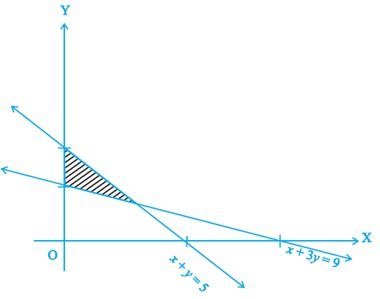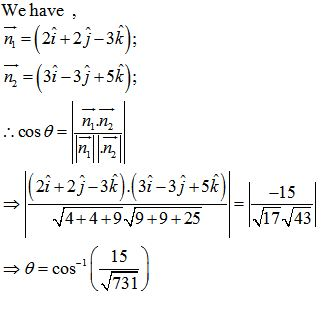# CBSE 12TH MATHEMATICS (Test 1)

Tag: cbse 12th mathematics
Q.1
A linear programming problem is one that is concerned with
A. finding the upper limits of a linear function of several variables
B. finding the lower limit of a linear function of several variables
C. finding the limiting values of a linear function of several variables
D. finding the optimal value (maximum or minimum) of a linear function of several variables
Explaination / Solution:

A linear programming problem is one that is concerned with finding the optimal value (maximum or minimum) of a linear function of several variables .

Workspace
Report
Q.2
In Corner point method for solving a linear programming problem the second step after finding the feasible region of the linear programming problem and determining its corner points is
A. Evaluate the objective function Z = ax + by at the mid points
B. None of these
C. Evaluate the objective function Z = ax + by at each corner point.
D. Evaluate the objective function Z = ax + by at the center point
Explaination / Solution:

In Corner point method for solving a linear programming problem the second step after finding the feasible region of the linear programming problem and determining its corner points is : To evaluate the objective function Z = ax + by at each corner point.

Workspace
Report
Q.3
Maximise Z = 3x + 4y subject to the constraints: x + y ≤ 4, x ≥ 0, y ≥ 0.
A. Maximum Z = 16 at (0, 4)
B. Maximum Z = 19 at (1, 5)
C. Maximum Z = 17 at (0, 5)
D. Maximum Z = 18 at (1, 4)
Explaination / Solution:

Objective function is Z = 3x + 4 y ……(1).
The given constraints are : x + y ≤ 4, x ≥ 0, y ≥ 0.

The corner points obtained by constructing the line x+ y= 4, are (0,0),(0,4) and (4,0).

 Corner points Z = 3x +4y O ( 0 ,0 ) Z = 3(0)+4(0) = 0 A ( 4 , 0 ) Z = 3(4) + 4 (0) =  12 B ( 0 , 4 ) Z = 3(0) + 4 ( 4) = 16 …( Max. )

therefore Z = 16 is maximum at ( 0 , 4 ) .

Workspace
Report
Q.4
Maximize Z = – x + 2y, subject to the constraints: x ≥ 3, x + y ≥ 5, x + 2y ≥ 6, y ≥ 0.
A. Maximum Z = 12 at (2, 6)
B. Maximum Z = 14 at (2, 6)
C. Z has no maximum value
D. Maximum Z = 10 at (2, 6)
Explaination / Solution:

Objective function is Z = - x + 2 y ……………………(1).
The given constraints are : x ≥ 3, x + y ≥ 5, x + 2y ≥ 6, y ≥ 0.

 Corner points Z =  - x + 2y D(6,0 ) -6 A(4,1) -2 B(3,2) 1

Here , the open half plane has points in common with the feasible region .
Therefore , Z has no maximum value.

Workspace
Report
Q.5
There are two types of fertilizers F1 and F2. F1 consists of 10% nitrogen and 6% phosphoric acid and F2 consists of 5% nitrogen and 10% phosphoric acid. After testing the soil conditions, a farmer finds that she needs atleast 14 kg of nitrogen and 14 kg of phosphoric acid for her crop. If F1 costs Rs 6/kg and F2 costsRs 5/kg, determine how much of each type of fertiliser should be used so that nutrient requirements are met at a minimum cost. What is the minimum cost?
A. 130 kg of fertilizer F1 and 80 kg of fertilizer F2; Minimum cost = Rs 1300
B. 120 kg of fertilizer F1 and 80 kg of fertilizer F2; Minimum cost = Rs 1200
C. 100 kg of fertilizer F1 and 80 kg of fertilizer F2; Minimum cost = Rs 1000
D. 110 kg of fertilizer F1 and 80 kg of fertilizer F2; Minimum cost = Rs 1100
Explaination / Solution:

Let number of kgs. of fertilizer F1 = x
And number of kgs. of fertilizer F2 = y
Therefore , the above L.P.P. is given as :
Minimise , Z = 6x +5y , subject to the constraints : 10/100 x + 5/100y ≥ 14 and 6/100x + 10/100y ≥ 14, i.e. 2 x + y ≥ 280 and 3x + 5y ≥ 700, x,y ≥ 0.,

 Corner points Z =6x +5 y A ( 0 , 280 ) 1400 D(700/3,0 ) 1400 B(100,80) 1000………….(Min.)

Corner points Z =6x +5 y A ( 0 , 280 ) 1400 D(700/3,0 ) 1400 B(100,80) 1000………….(Min.) Here Z = 1000 is minimum.
i.e. 100 kg of fertilizer F1 and 80 kg of fertilizer F2; Minimum cost = Rs 1000.

Workspace
Report
Q.6

The feasible region for a LPP is shown in Figure. Find the minimum value of Z = 11x + 7y.A. 19
B. 20
C. 21
D. 22
Explaination / Solution:

 Corner points Z = 11x +7 y (0, 5 ) 35 (0,3) 21…………………(min.) (3,2 ) 47

Hence the minimum value is 21

Workspace
Report
Q.7
Vector equation of a line that passes through the given point whose position vector is     and parallel to a given vector  is
A.B.C.D.Explaination / Solution:

Vector equation of a line that passes through the given point whose position vector is    and parallel to a given vector     is given by :Workspace
Report
Q.8
Find the equation of the line which passes through the point (1, 2, 3) and is parallel to the vector.

A.  +
B.  +
C.  +
D.  +
Explaination / Solution:

The equation of the line which passes through the point (1, 2, 3) and is parallel to the vector
, let vector  and vector
the equation of line is :
.
Workspace
Report
Q.9
Equation of a plane which is at a distance d from the origin and the direction cosines of the normal to the plane are l, m, n is.
A. – lx + my + nz = d
B. lx – my + nz = d
C. lx + my + nz = – d
D. lx + my + nz = d
Explaination / Solution:

In Cartesian co – ordinate system Equation of a plane which is at a distance d from the origin and the direction cosines of the normal to the plane are l, m, n is given by : lx + my + nz = d.

Workspace
Report
Q.10
Find the angle between the planes whose vector equations areA. cos1(15731)
B. sin1(15731)
C. tan1(15731)
D. cot1(15731)